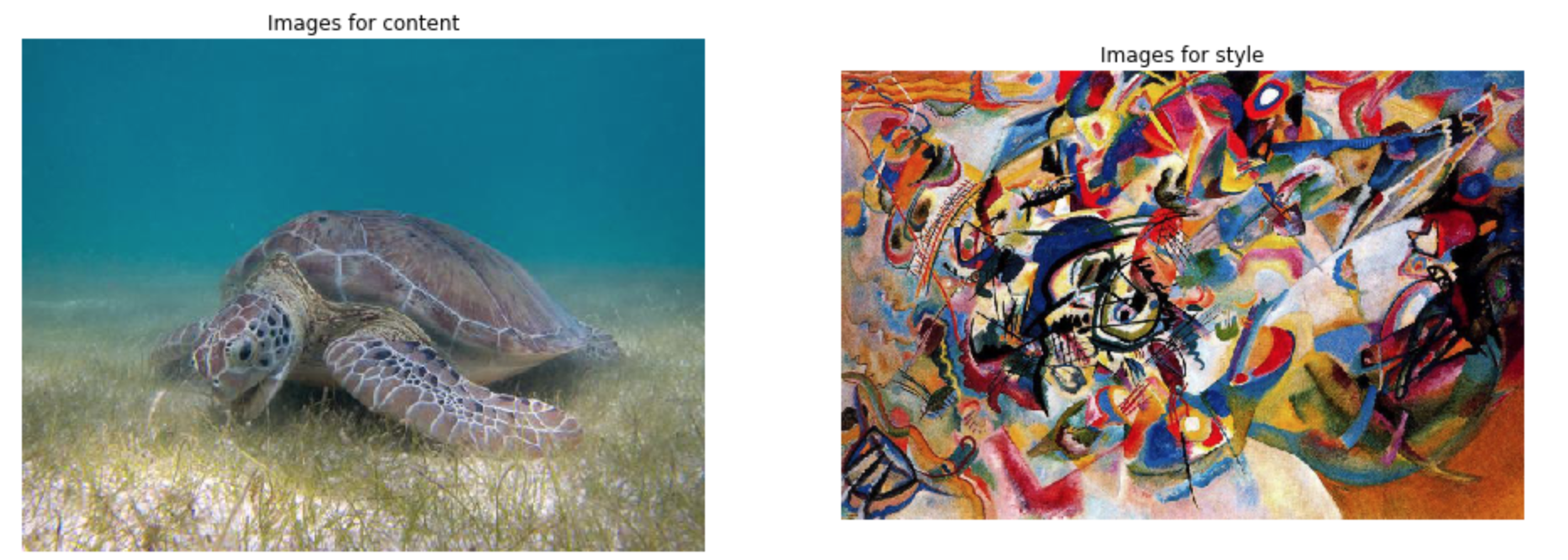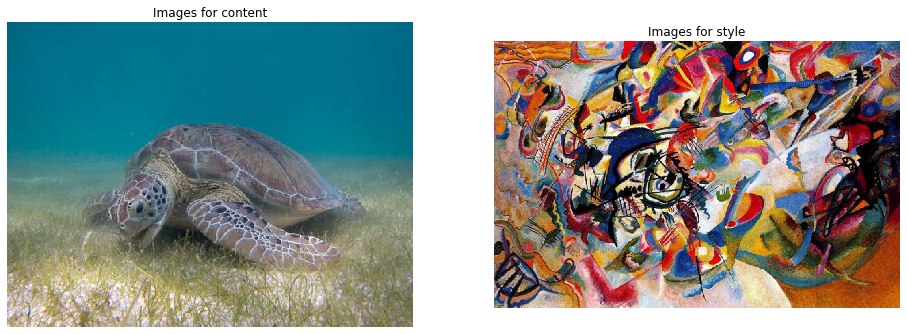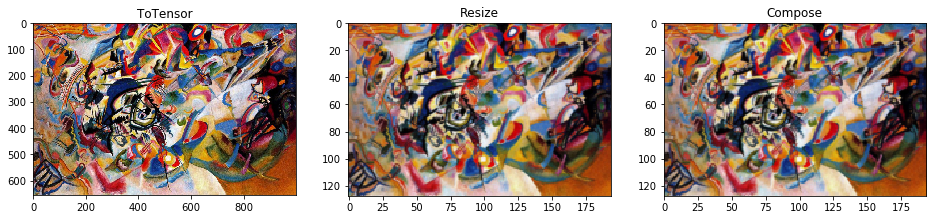# Style Transfer using Pytorch (Part 1)

## Goal¶

This post aims to follow the tutorial NEURAL TRANSFER USING PYTORCH step-by-step. Part 1 is about image loading. The following images for content and style are loaded as PyTorch tensor.Reference

## Libraries¶

In :
# Torch & Tensorflow
import torch
import tensorflow as tf

# Visualization
from PIL import Image
import torchvision.transforms as transforms
import matplotlib.pyplot as plt
%matplotlib inline


## Configuration¶

In :
device = torch.device("cuda" if torch.cuda.is_available() else "cpu")


In :
d_path = {}

In :
fig, axes = plt.subplots(1, 2, figsize=(16, 8))
for i, key in enumerate(d_path.keys()):
axes[i].imshow(img);
axes[i].axis('off');
axes[i].set_title(f'Images for {key}')## Create an image loader for PyTorch¶

To use PyTorch, images need to be loaded as tensor through the image loader.

In :
# desired size of the output image
imsize = 512 if torch.cuda.is_available() else 128  # use small size if no gpu

transforms.Resize(imsize),  # scale imported image
transforms.ToTensor()])  # transform it into a torch tensor

image = Image.open(image_name)

# fake batch dimension required to fit network's input dimensions
return image.to(device, torch.float)

In :
style_img = image_loader(d_path['content'])


### Plot tensor images as PIL image¶

In :
unloader = transforms.ToPILImage()  # reconvert into PIL image

def imshow(tensor, title=None):
image = tensor.cpu().clone()  # we clone the tensor to not do changes on it
image = image.squeeze(0)      # remove the fake batch dimension
plt.imshow(image)
if title is not None:
plt.title(title)
plt.pause(0.001) # pause a bit so that plots are updated


### Check transform process¶

In :
totensor = transforms.ToTensor()
scale = transforms.Resize(128)
composed = transforms.Compose([transforms.Resize(128),
transforms.ToTensor()])

# Apply each of the above transforms on sample.
fig = plt.figure(figsize=(16, 6))
print(f'Original image size: {img.size}')
for i, tsfrm in enumerate([totensor, scale, composed]):
transformed_sample = tsfrm(img)
if i != 1:
transformed_sample = transformed_sample.cpu().clone().squeeze(0)

ax = plt.subplot(1, 3, i + 1)
ax.set_title(type(tsfrm).__name__)
ax.imshow(transformed_sample)

Original image size: (1000, 657)In [ ]: